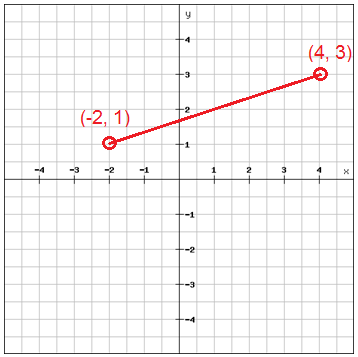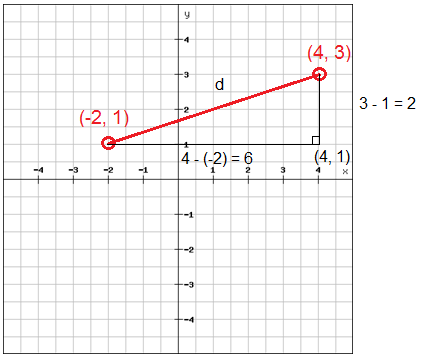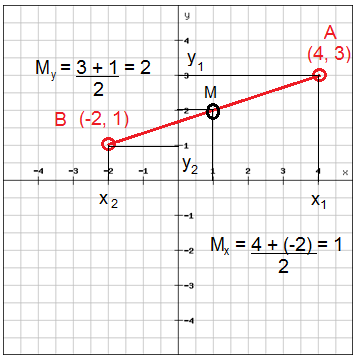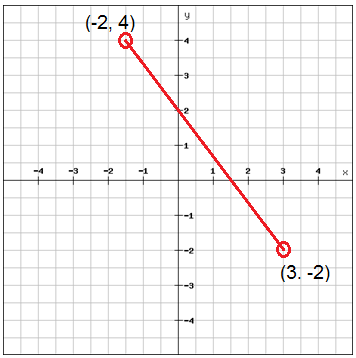# The distance and midpoint formulas

The distance formula is used to find the distance between two points in the coordinate plane. We'll explain this using an example belowWe want to calculate the distance between the two points (-2, 1) and (4, 3). We could see the line drawn between these two points is the hypotenuse of a right triangle. The legs of this triangle would be parallel to the axes which mean that we can measure the length of the legs easily.We'll get the length of the distance d by using the Pythagorean Theorem

$d^{2}=2^{2}+6^{2}=4+36=40$

$d=\sqrt{40}\approx 6.32$

This method can be used to determine the distance between any two points in a coordinate plane and is summarized in the distance formula

$d=\sqrt{\left ( x_{2}-x_{1} \right )^{2}+\left ( y_{2}-y_{1} \right )^{2}}$

The point that is at the same distance from two points A (x1, y1) and B (x2, y2) on a line is called the midpoint. You calculate the midpoint using the midpoint formula

$m =\left ( \frac{x_{1}+x_{2}}{2} \right ),\: \: \left ( \frac{y_{1}+y_{2}}{2} \right )$

We can use the example above to illustrate this$m =\left ( \frac{4+(-2)}{2} \right ),\: \: \left ( \frac{3+1}{2} \right )=$

$=\left ( \frac{2}{2} \right ),\: \: \left ( \frac{4}{2} \right )=\begin{pmatrix} 1,\: 2 \end{pmatrix}$

## Video lesson

Calculate the distance between the two points